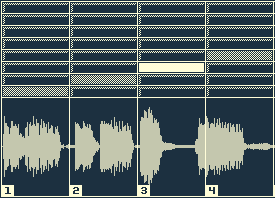# Slicer with waveform displaySlicer with waveform display

This slicer works the same as the previous one. Only difference is that is uses eight slices instead of sixteen.

The new stuff is in the interface: Waveforms to see what’s playing. And nicer to look at as well.

To use this app you can click the buttons as before to select a slice to play, and now you will see the waveform change to represent the slice.

The second way to change the slices is to click and drag on the waveforms itself. Drag up for later slices and down for earlier ones. Drag all the way down for an empty slice and silence.

Here it is! The music is from a track by Flying Lotus. I don’t know which one exactly: It’s track nr. 23 from a collection called “38 Cartoons”, but I couldn’t find any information about it online.

## How to get an image from MP3 sound data

The way I did it is first to calculate how many samples of a slice represent one pixel of the image, then get the biggest amplitude of those samples. That will be a number between 0 and 1. Then multiply it by half of the images height to get the size of the line to draw. And finally draw the line to a BitMapData object.

It generates a simplified waveform: The positive and negative amplitudes are the same and it’s only the left channel. But it’s good enough for this purpose. It’s a good impression of the waveform.

Here is the main part of the code:

```var mp3NumSamples : Number = mMp3Loop.extract(new ByteArray(), (41000 * 60 * 60)) - mMp3StartOffset;
mMp3NumSamplesPerSlice = Math.round(mp3NumSamples / Model.NUM_SLICES);
mBPM = TimeConversion.numSamplesToBPM(mp3NumSamples, 2);

var sampleIndex : int = mMp3StartOffset;
var imageWidth : int = Model.BUTTON_WIDTH;
var samplesPerPixel : int = mMp3NumSamplesPerSlice / imageWidth;

// loop through the slices
for(var i : int = 0;i < Model.NUM_SLICES; ++i)
{
var byteArray : ByteArray = new ByteArray();
var numSamples : int = mMp3Loop.extract(byteArray, mMp3NumSamplesPerSlice, sampleIndex);
var position : int = 0;
var lineMiddle : int = (Model.DISPLAY_HEIGHT / 2);

byteArray.position = 0;

mMp3WaveformData[i] = new BitmapData(Model.BUTTON_WIDTH, Model.DISPLAY_HEIGHT, true, 0xFF0000);
var bitmapData : BitmapData = mMp3WaveformData[i];

// loop through the pixels of a waveform image's width
for(var j : int = 0;j < imageWidth; ++j)
{
var waveHeight : Number = 0.0;

// get maximum of all samples within pixel
for(var k : int = 0; k < samplesPerPixel ; ++k)
{
if(position < numSamples)
{
var l : Number = byteArray.readFloat();
}
else
{
l = 0;
}

waveHeight = Math.max(waveHeight, Math.abs(l));
position ++;
}

waveHeight *= lineMiddle;
var start : int = lineMiddle - waveHeight;
var end : int = lineMiddle + waveHeight;

// draw to bitmapdata
for(k = start; k < end ; ++k)
{
bitmapData.setPixel32(j, k, 0xFFFFFFCC);
}
}

sampleIndex += mMp3NumSamplesPerSlice;
}
```